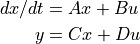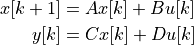# control.matlab.ss¶

control.matlab.ss(A, B, C, D[, dt])[source]

Create a state space system.

The function accepts either 1, 2, 4 or 5 parameters:

`ss(sys)`

Convert a linear system into space system form. Always creates a new system, even if sys is already a state space system.

`ss(updfcn, outfcn)`

Create a nonlinear input/output system with update function `updfcn` and output function `outfcn`. See `NonlinearIOSystem` for more information.

`ss(A, B, C, D)`

Create a state space system from the matrices of its state and output equations:`ss(A, B, C, D, dt)`

Create a discrete-time state space system from the matrices of its state and output equations:The matrices can be given as array like data types or strings. Everything that the constructor of `numpy.matrix` accepts is permissible here too.

`ss(args, inputs=['u1', ..., 'up'], outputs=['y1', ..., 'yq'], states=['x1', ..., 'xn'])`

Create a system with named input, output, and state signals.

Parameters
• sys (StateSpace or TransferFunction) – A linear system.

• A (array_like or string) – System, control, output, and feed forward matrices.

• B (array_like or string) – System, control, output, and feed forward matrices.

• C (array_like or string) – System, control, output, and feed forward matrices.

• D (array_like or string) – System, control, output, and feed forward matrices.

• dt (None, True or float, optional) – System timebase. 0 (default) indicates continuous time, True indicates discrete time with unspecified sampling time, positive number is discrete time with specified sampling time, None indicates unspecified timebase (either continuous or discrete time).

• inputs (str, or list of str, optional) – List of strings that name the individual signals. If this parameter is not given or given as None, the signal names will be of the form s[i] (where s is one of u, y, or x). See `InputOutputSystem` for more information.

• outputs (str, or list of str, optional) – List of strings that name the individual signals. If this parameter is not given or given as None, the signal names will be of the form s[i] (where s is one of u, y, or x). See `InputOutputSystem` for more information.

• states (str, or list of str, optional) – List of strings that name the individual signals. If this parameter is not given or given as None, the signal names will be of the form s[i] (where s is one of u, y, or x). See `InputOutputSystem` for more information.

• name (string, optional) – System name (used for specifying signals). If unspecified, a generic name <sys[id]> is generated with a unique integer id.

Returns

out – Linear input/output system.

Return type

`LinearIOSystem`

Raises

ValueError – If matrix sizes are not self-consistent.

```>>> G = ct.ss([[-1, -2], [3, -4]], [, ], [[6, 8]], [])
```>>> sys_tf = ct.tf([2.], [1., 3])